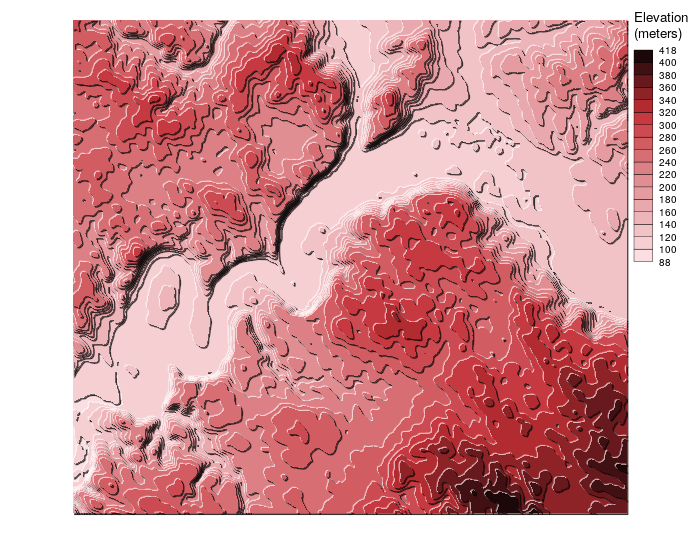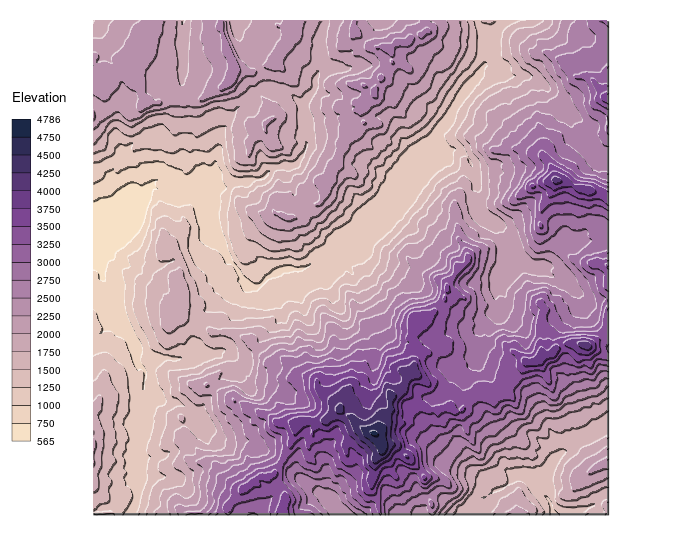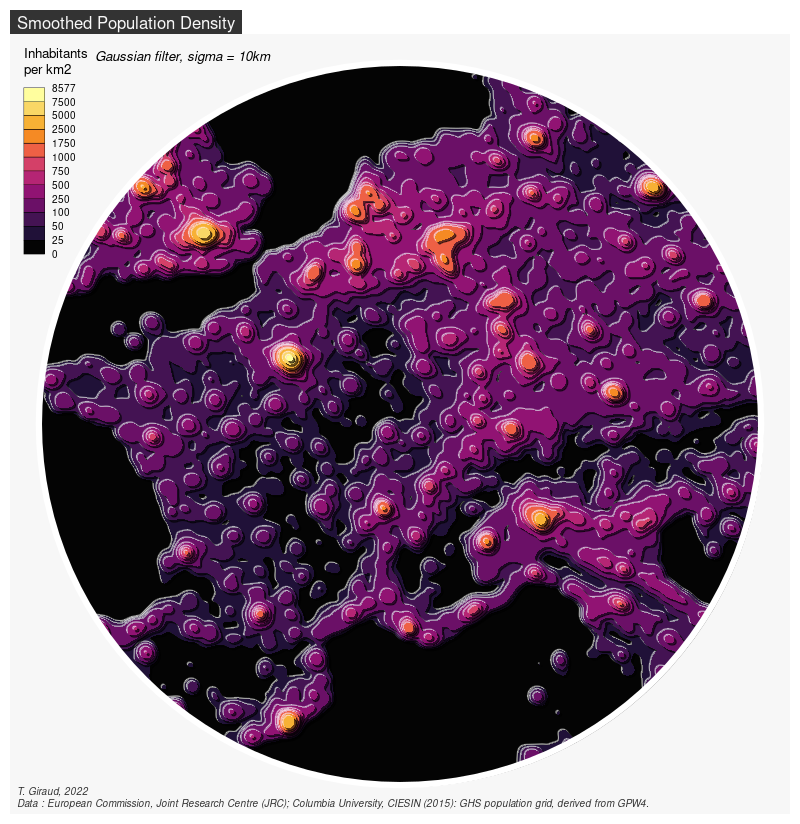# TanakaAlso called “relief contours method”, “illuminated contour method” or “shaded contour lines method”, the Tanaka method1 enhances the representation of topography on a map by using shaded contour lines. The result is a 3D-like map.

This package is a simplified implementation of the Tanaka method, north-west white contours represent illuminated topography and south-east black contours represent shaded topography. Even if the results are quite satisfactory, a more refined method could be used based on the Kennelly and Kimerling’s paper2.

`tanaka` is a small package with two functions:

• `tanaka()` uses a `raster` object and displays the map directly;
• `tanaka_contour()` builds the isopleth polygon layer.

The contour lines creation relies on `isoband`, spatial manipulation and display rely on `sf`.

## Installation

• From CRAN
``install.packages("tanaka")``
• Development version on GitHub
``````require(remotes)
install_github("rCarto/tanaka")``````

## Demo

• This example is based on the dataset shipped within the package.
``````library(tanaka)
library(raster)
ras <- raster(system.file("grd/elev.grd", package = "tanaka"))
tanaka(ras, breaks = seq(80,400,20),
legend.pos = "topright", legend.title = "Elevation\n(meters)")````````````library(tanaka)
library(elevatr)
# use elevatr to get elevation data
ras <- get_elev_raster(locations = data.frame(x = c(6.7, 7), y = c(45.8,46)),
z = 10, prj = "+init=epsg:4326", clip = "locations")
# custom color palette
cols <- c("#F7E1C6", "#EED4C1", "#E5C9BE", "#DCBEBA", "#D3B3B6", "#CAA8B3",
"#C19CAF", "#B790AB", "#AC81A7", "#A073A1", "#95639D", "#885497",
"#7C4692", "#6B3D86", "#573775", "#433266", "#2F2C56", "#1B2847")
# display the map
tanaka(ras, breaks = seq(500,4800,250), col = cols)````````````library(raster)
library(sf)
library(cartography)
library(tanaka)

temp <- tempfile()
data_url <- "http://cidportal.jrc.ec.europa.eu/ftp/jrc-opendata/GHSL/GHS_POP_GPW4_GLOBE_R2015A/GHS_POP_GPW42015_GLOBE_R2015A_54009_1k/V1-0/GHS_POP_GPW42015_GLOBE_R2015A_54009_1k_v1_0.zip"
unzip(temp, exdir = "pop")
pop2015 <- raster("pop/GHS_POP_GPW42015_GLOBE_R2015A_54009_1k_v1_0/GHS_POP_GPW42015_GLOBE_R2015A_54009_1k_v1_0.tif")
center <- st_as_sf(data.frame(x=425483.8, y=5608290),
coords=(c("x","y")), crs = st_crs(pop2015))
center <- st_buffer(center, dist = 800000)
ras <- crop(pop2015, st_bbox(center)[c(1,3,2,4)])
mat <- focalWeight(x = ras, d = c(10000), type = "Gauss")
rassmooth <- focal(x = ras, w = mat, fun = sum, pad = TRUE, padValue = 30)
bks <- c(0,25,50,100,250,500,750,1000,1750,2500,5000, 7500,10000)
png(filename = "circle.png", width = 800, height = 700, res = 100)
par(mar = c(0,0,1.2,0))
tanaka(x = rassmooth,
breaks = bks,
col = hcl.colors(n = 12, palette = "Inferno"),
shift = 2500,
legend.pos = "topleft",
legend.title = "Inhabitants\nper km2")
plot(st_geometry(center), add = T, border = "white", lwd = 6)
layoutLayer(title = "Smoothed Population Density",
author = 'Data : European Commission, Joint Research Centre (JRC); Columbia University, CIESIN (2015): GHS population grid, derived from GPW4.',
sources = 'T. Giraud, 2019', scale = F, frame = F, tabtitle = TRUE)
text(-374516.2 ,6408290.0, "Gaussian smoothing, sigma = 10km", adj = 0, font = 3, cex = .8 )
dev.off()``````## Alternative Package

The `metR` package allows to draw Tanaka contours with ggplot2.

1: Tanaka, K. (1950). The relief contour method of representing topography on maps. Geographical Review, 40(3), 444-456.
2: Kennelly, P., & Kimerling, A. J. (2001). Modifications of Tanaka’s illuminated contour method. Cartography and Geographic Information Science, 28(2), 111-123.